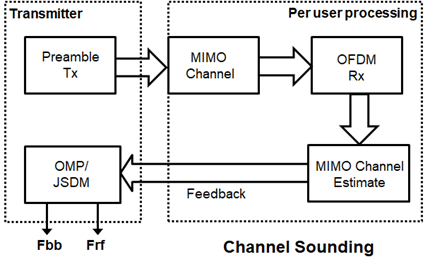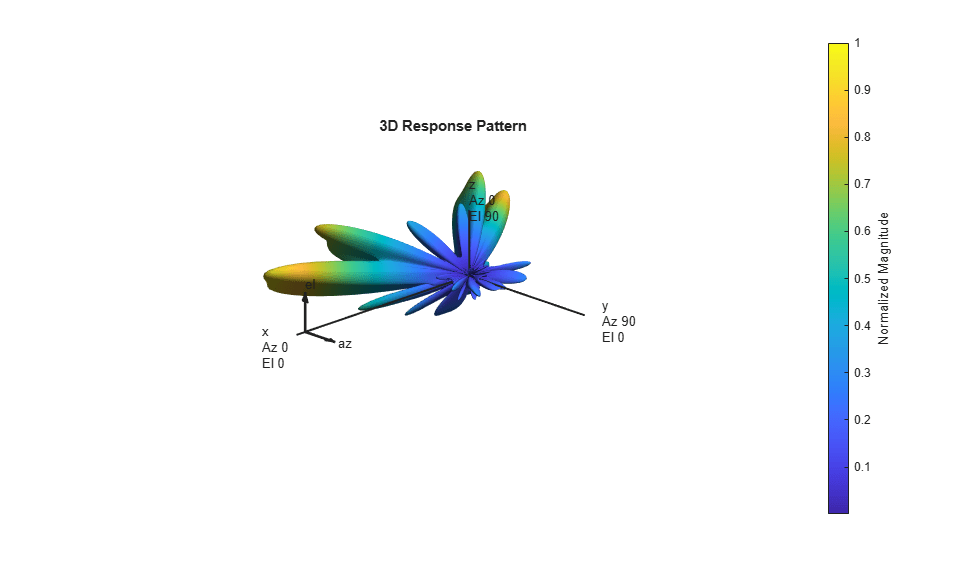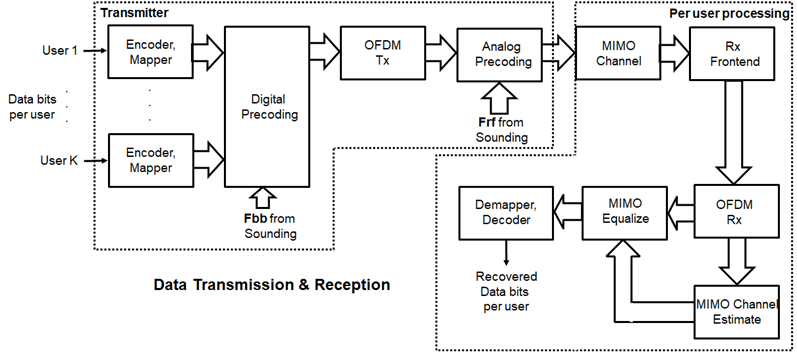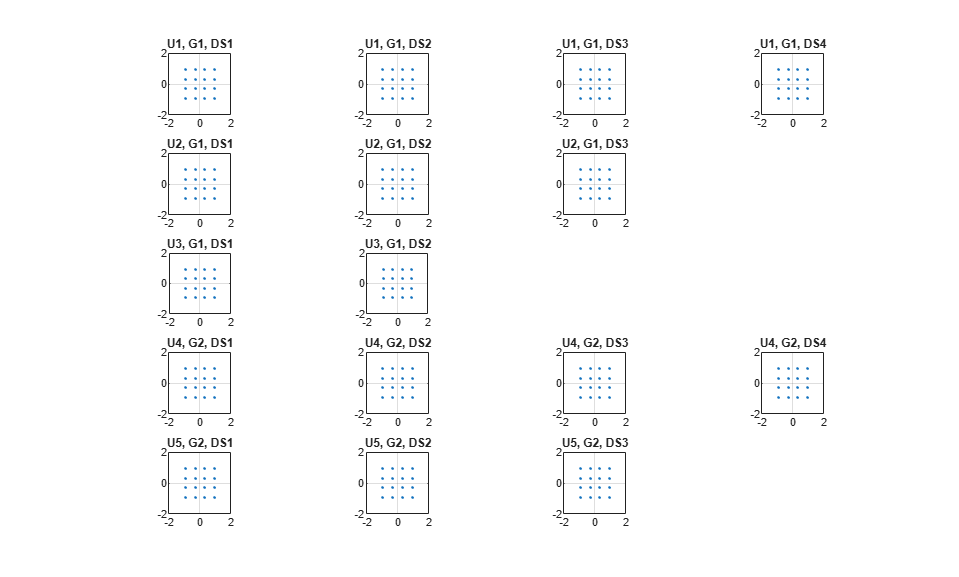# Massive MIMO Hybrid Beamforming

This example shows how hybrid beamforming is employed at the transmit end of a massive MIMO communications system, using techniques for both multi-user and single-user systems. The example employs full channel sounding for determining the channel state information at the transmitter. It partitions the required precoding into digital baseband and analog RF components, using different techniques for multi-user and single-user systems. Simplified all-digital receivers recover the multiple transmitted data streams to highlight the common figures of merit for a communications system, namely, EVM, and BER.

The example employs a scattering-based spatial channel model which accounts for the transmit/receive spatial locations and antenna patterns. A simpler static-flat MIMO channel is also offered for link validation purposes.

The example requires Communications Toolbox™ and Phased Array System Toolbox™.

### Introduction

The ever-growing demand for high data rate and more user capacity increases the need to use the available spectrum more efficiently. Multi-user MIMO (MU-MIMO) improves the spectrum efficiency by allowing a base station (BS) transmitter to communicate simultaneously with multiple mobile stations (MS) receivers using the same time-frequency resources. Massive MIMO allows the number of BS antenna elements to be on the order of tens or hundreds, thereby also increasing the number of data streams in a cell to a large value.

The next generation, 5G, wireless systems use millimeter wave (mmWave) bands to take advantage of their wider bandwidth. The 5G systems also deploy large scale antenna arrays to mitigate severe propagation loss in the mmWave band.

Compared to current wireless systems, the wavelength in the mmWave band is much smaller. Although this allows an array to contain more elements within the same physical dimension, it becomes much more expensive to provide one transmit-receive (TR) module, or an RF chain, for each antenna element. Hybrid transceivers are a practical solution as they use a combination of analog beamformers in the RF and digital beamformers in the baseband domains, with fewer RF chains than the number of transmit elements [ 1 ].

This example uses a multi-user MIMO-OFDM system to highlight the partitioning of the required precoding into its digital baseband and RF analog components at the transmitter end. Building on the system highlighted in the MIMO-OFDM Precoding with Phased Arrays (Phased Array System Toolbox) example, this example shows the formulation of the transmit-end precoding matrices and their application to a MIMO-OFDM system.

```s = rng(67); % Set RNG state for repeatability ```

### System Parameters

Define system parameters for the example. Modify these parameters to explore their impact on the system.

```% Multi-user system with single/multiple streams per user prm.numUsers = 4; % Number of users prm.numSTSVec = [3 2 1 2]; % Number of independent data streams per user prm.numSTS = sum(prm.numSTSVec); % Must be a power of 2 prm.numTx = prm.numSTS*8; % Number of BS transmit antennas (power of 2) prm.numRx = prm.numSTSVec*4; % Number of receive antennas, per user (any >= numSTSVec) % Each user has the same modulation prm.bitsPerSubCarrier = 4; % 2: QPSK, 4: 16QAM, 6: 64QAM, 8: 256QAM prm.numDataSymbols = 10; % Number of OFDM data symbols % MS positions: assumes BS at origin % Angles specified as [azimuth;elevation] degrees % az in range [-180 180], el in range [-90 90], e.g. [45;0] maxRange = 1000; % all MSs within 1000 meters of BS prm.mobileRanges = randi([1 maxRange],1,prm.numUsers); prm.mobileAngles = [rand(1,prm.numUsers)*360-180; ... rand(1,prm.numUsers)*180-90]; prm.fc = 28e9; % 28 GHz system prm.chanSRate = 100e6; % Channel sampling rate, 100 Msps prm.ChanType = 'Scattering'; % Channel options: 'Scattering', 'MIMO' prm.NFig = 8; % Noise figure (increase to worsen, 5-10 dB) prm.nRays = 500; % Number of rays for Frf, Fbb partitioning ```

Define OFDM modulation parameters used for the system.

```prm.FFTLength = 256; prm.CyclicPrefixLength = 64; prm.numCarriers = 234; prm.NullCarrierIndices = [1:7 129 256-5:256]'; % Guards and DC prm.PilotCarrierIndices = [26 54 90 118 140 168 204 232]'; nonDataIdx = [prm.NullCarrierIndices; prm.PilotCarrierIndices]; prm.CarriersLocations = setdiff((1:prm.FFTLength)', sort(nonDataIdx)); numSTS = prm.numSTS; numTx = prm.numTx; numRx = prm.numRx; numSTSVec = prm.numSTSVec; codeRate = 1/3; % same code rate per user numTails = 6; % number of termination tail bits prm.numFrmBits = numSTSVec.*(prm.numDataSymbols*prm.numCarriers* ... prm.bitsPerSubCarrier*codeRate)-numTails; prm.modMode = 2^prm.bitsPerSubCarrier; % Modulation order % Account for channel filter delay numPadSym = 3; % number of symbols to zeropad prm.numPadZeros = numPadSym*(prm.FFTLength+prm.CyclicPrefixLength); ```

Define transmit and receive arrays and positional parameters for the system.

```prm.cLight = physconst('LightSpeed'); prm.lambda = prm.cLight/prm.fc; % Get transmit and receive array information [isTxURA,expFactorTx,isRxURA,expFactorRx] = helperArrayInfo(prm,true); % Transmit antenna array definition % Array locations and angles prm.posTx = [0;0;0]; % BS/Transmit array position, [x;y;z], meters if isTxURA % Uniform Rectangular array txarray = phased.PartitionedArray(... 'Array',phased.URA([expFactorTx numSTS],0.5*prm.lambda),... 'SubarraySelection',ones(numSTS,numTx),'SubarraySteering','Custom'); else % Uniform Linear array txarray = phased.ULA(numTx, 'ElementSpacing',0.5*prm.lambda, ... 'Element',phased.IsotropicAntennaElement('BackBaffled',false)); end prm.posTxElem = getElementPosition(txarray)/prm.lambda; spLoss = zeros(prm.numUsers,1); prm.posRx = zeros(3,prm.numUsers); for uIdx = 1:prm.numUsers % Receive arrays if isRxURA(uIdx) % Uniform Rectangular array rxarray = phased.PartitionedArray(... 'Array',phased.URA([expFactorRx(uIdx) numSTSVec(uIdx)], ... 0.5*prm.lambda),'SubarraySelection',ones(numSTSVec(uIdx), ... numRx(uIdx)),'SubarraySteering','Custom'); prm.posRxElem = getElementPosition(rxarray)/prm.lambda; else if numRx(uIdx)>1 % Uniform Linear array rxarray = phased.ULA(numRx(uIdx), ... 'ElementSpacing',0.5*prm.lambda, ... 'Element',phased.IsotropicAntennaElement); prm.posRxElem = getElementPosition(rxarray)/prm.lambda; else rxarray = phased.IsotropicAntennaElement; prm.posRxElem = [0; 0; 0]; % LCS end end % Mobile positions [xRx,yRx,zRx] = sph2cart(deg2rad(prm.mobileAngles(1,uIdx)), ... deg2rad(prm.mobileAngles(2,uIdx)), ... prm.mobileRanges(uIdx)); prm.posRx(:,uIdx) = [xRx;yRx;zRx]; [toRxRange,toRxAng] = rangeangle(prm.posTx,prm.posRx(:,uIdx)); spLoss(uIdx) = fspl(toRxRange,prm.lambda); end ```

### Channel State Information

For a spatially multiplexed system, availability of channel information at the transmitter allows for precoding to be applied to maximize the signal energy in the direction and channel of interest. Under the assumption of a slowly varying channel, this is facilitated by sounding the channel first. The BS sounds the channel by using a reference transmission, that the MS receiver uses to estimate the channel. The MS transmits the channel estimate information back to the BS for calculation of the precoding needed for the subsequent data transmission.

The following schematic shows the processing for the channel sounding modeled.For the chosen MIMO system, a preamble signal is sent over all transmitting antenna elements, and processed at the receiver accounting for the channel. The receiver antenna elements perform pre-amplification, OFDM demodulation, and frequency domain channel estimation for all links.

```% Generate the preamble signal prm.numSTS = numTx; % set to numTx to sound out all channels preambleSig = helperGenPreamble(prm); % Transmit preamble over channel prm.numSTS = numSTS; % keep same array config for channel [rxPreSig,chanDelay] = helperApplyMUChannel(preambleSig,prm,spLoss); % Channel state information feedback hDp = cell(prm.numUsers,1); prm.numSTS = numTx; % set to numTx to estimate all links for uIdx = 1:prm.numUsers % Front-end amplifier gain and thermal noise rxPreAmp = phased.ReceiverPreamp( ... 'Gain',spLoss(uIdx), ... % account for path loss 'NoiseFigure',prm.NFig,'ReferenceTemperature',290, ... 'SampleRate',prm.chanSRate); rxPreSigAmp = rxPreAmp(rxPreSig{uIdx}); % scale power for used sub-carriers rxPreSigAmp = rxPreSigAmp * (sqrt(prm.FFTLength - ... length(prm.NullCarrierIndices))/prm.FFTLength); % OFDM demodulation rxOFDM = ofdmdemod(rxPreSigAmp(chanDelay(uIdx)+1: ... end-(prm.numPadZeros-chanDelay(uIdx)),:),prm.FFTLength, ... prm.CyclicPrefixLength,prm.CyclicPrefixLength, ... prm.NullCarrierIndices,prm.PilotCarrierIndices); % Channel estimation from preamble % numCarr, numTx, numRx hDp{uIdx} = helperMIMOChannelEstimate(rxOFDM(:,1:numTx,:),prm); end ```

For a multi-user system, the channel estimate is fed back from each MS, and used by the BS to determine the precoding weights. The example assumes perfect feedback with no quantization or implementation delays.

### Hybrid Beamforming

The example uses the orthogonal matching pursuit (OMP) algorithm [ 3 ] for a single-user system and the joint spatial division multiplexing (JSDM) technique [ 2, 4 ] for a multi-user system, to determine the digital baseband `Fbb` and RF analog `Frf` precoding weights for the selected system configuration.

For a single-user system, the OMP partitioning algorithm is sensitive to the array response vectors `At`. Ideally, these response vectors account for all the scatterers seen by the channel, but these are unknown for an actual system and channel realization, so a random set of rays within a 3-dimensional space to cover as many scatterers as possible is used. The `prm.nRays` parameter specifies the number of rays.

For a multi-user system, JSDM groups users with similar transmit channel covariance together and suppresses the inter-group interference by an analog precoder based on the block diagonalization method [ 5 ]. Here each user is assigned to be in its own group, thereby leading to no reduction in the sounding or feedback overhead.

```% Calculate the hybrid weights on the transmit side if prm.numUsers==1 % Single-user OMP % Spread rays in [az;el]=[-180:180;-90:90] 3D space, equal spacing % txang = [-180:360/prm.nRays:180; -90:180/prm.nRays:90]; txang = [rand(1,prm.nRays)*360-180;rand(1,prm.nRays)*180-90]; % random At = steervec(prm.posTxElem,txang); AtExp = complex(zeros(prm.numCarriers,size(At,1),size(At,2))); for carrIdx = 1:prm.numCarriers AtExp(carrIdx,:,:) = At; % same for all sub-carriers end % Orthogonal matching pursuit hybrid weights [Fbb,Frf] = omphybweights(hDp{1},numSTS,numSTS,AtExp); v = Fbb; % set the baseband precoder (Fbb) % Frf is same across subcarriers for flat channels mFrf = permute(mean(Frf,1),[2 3 1]); else % Multi-user Joint Spatial Division Multiplexing [Fbb,mFrf] = helperJSDMTransmitWeights(hDp,prm); % Multi-user baseband precoding % Pack the per user CSI into a matrix (block diagonal) steeringMatrix = zeros(prm.numCarriers,sum(numSTSVec),sum(numSTSVec)); for uIdx = 1:prm.numUsers stsIdx = sum(numSTSVec(1:uIdx-1))+(1:numSTSVec(uIdx)); steeringMatrix(:,stsIdx,stsIdx) = Fbb{uIdx}; % Nst-by-Nsts-by-Nsts end v = permute(steeringMatrix,[1 3 2]); end % Transmit array pattern plots if isTxURA % URA element response for the first subcarrier pattern(txarray,prm.fc,-180:180,-90:90,'Type','efield', ... 'ElementWeights',mFrf.'*squeeze(v(1,:,:)), ... 'PropagationSpeed',prm.cLight); else % ULA % Array response for first subcarrier wts = mFrf.'*squeeze(v(1,:,:)); pattern(txarray,prm.fc,-180:180,-90:90,'Type','efield', ... 'Weights',wts(:,1),'PropagationSpeed',prm.cLight); end prm.numSTS = numSTS; % revert back for data transmission ```For the wideband OFDM system modeled, the analog weights, `mFrf`, are the averaged weights over the multiple subcarriers. The array response pattern shows distinct data streams represented by the stronger lobes. These lobes indicate the spread or separability achieved by beamforming. The Introduction to Hybrid Beamforming (Phased Array System Toolbox) example compares the patterns realized by the optimal, fully digital approach, with those realized from the selected hybrid approach, for a single-user system.

### Data Transmission

The example models an architecture where each data stream maps to an individual RF chain and each antenna element is connected to each RF chain. This is shown in the following diagram.Next, we configure the system's data transmitter. This processing includes channel coding, bit mapping to complex symbols, splitting of the individual data stream to multiple transmit streams, baseband precoding of the transmit streams, OFDM modulation with pilot mapping and RF analog beamforming for all the transmit antennas employed.

```% Convolutional encoder encoder = comm.ConvolutionalEncoder( ... 'TrellisStructure',poly2trellis(7,[133 171 165]), ... 'TerminationMethod','Terminated'); txDataBits = cell(prm.numUsers, 1); gridData = complex(zeros(prm.numCarriers,prm.numDataSymbols,numSTS)); for uIdx = 1:prm.numUsers % Generate mapped symbols from bits per user txDataBits{uIdx} = randi([0,1],prm.numFrmBits(uIdx),1); encodedBits = encoder(txDataBits{uIdx}); % Bits to QAM symbol mapping mappedSym = qammod(encodedBits,prm.modMode,'InputType','bit', ... 'UnitAveragePower',true); % Map to layers: per user, per symbol, per data stream stsIdx = sum(numSTSVec(1:(uIdx-1)))+(1:numSTSVec(uIdx)); gridData(:,:,stsIdx) = reshape(mappedSym,prm.numCarriers, ... prm.numDataSymbols,numSTSVec(uIdx)); end % Apply precoding weights to the subcarriers, assuming perfect feedback preData = complex(zeros(prm.numCarriers,prm.numDataSymbols,numSTS)); for symIdx = 1:prm.numDataSymbols for carrIdx = 1:prm.numCarriers Q = squeeze(v(carrIdx,:,:)); normQ = Q * sqrt(numTx)/norm(Q,'fro'); preData(carrIdx,symIdx,:) = squeeze(gridData(carrIdx,symIdx,:)).' ... * normQ; end end % Multi-antenna pilots pilots = helperGenPilots(prm.numDataSymbols,numSTS); % OFDM modulation of the data txOFDM = ofdmmod(preData,prm.FFTLength,prm.CyclicPrefixLength,... prm.NullCarrierIndices,prm.PilotCarrierIndices,pilots); % scale power for used sub-carriers txOFDM = txOFDM * (prm.FFTLength/ ... sqrt((prm.FFTLength-length(prm.NullCarrierIndices)))); % Generate preamble with the feedback weights and prepend to data preambleSigD = helperGenPreamble(prm,v); txSigSTS = [preambleSigD;txOFDM]; % RF beamforming: Apply Frf to the digital signal % Each antenna element is connected to each data stream txSig = txSigSTS*mFrf; ```

For the selected, fully connected RF architecture, each antenna element uses `prm.numSTS` phase shifters, as given by the individual columns of the `mFrf` matrix.

The processing for the data transmission and reception modeled is shown below.### Signal Propagation

The example offers an option for spatial MIMO channel and a simpler static-flat MIMO channel for validation purposes.

The scattering model uses a single-bounce ray tracing approximation with a parametrized number of scatterers. For this example, the number of scatterers is set to 100. The 'Scattering' option models the scatterers placed randomly within a sphere around the receiver, similar to the one-ring model [ 6 ].

The channel models allow path-loss modeling and both line-of-sight (LOS) and non-LOS propagation conditions. The example assumes non-LOS propagation and isotropic antenna element patterns with linear or rectangular geometry.

```% Apply a spatially defined channel to the transmit signal [rxSig,chanDelay] = helperApplyMUChannel(txSig,prm,spLoss,preambleSig); ```

The same channel is used for both sounding and data transmission. The data transmission has a longer duration and is controlled by the number of data symbols parameter, `prm.numDataSymbols`. The channel evolution between the sounding and transmission stages is modeled by prepending the preamble signal to the data signal. The preamble primes the channel to a valid state for the data transmission, and is ignored from the channel output.

For a multi-user system, independent channels per user are modeled.

### Receive Amplification and Signal Recovery

The receiver modeled per user compensates for the path loss by amplification and adds thermal noise. Like the transmitter, the receiver used in a MIMO-OFDM system contains many stages including OFDM demodulation, MIMO equalization, QAM demapping, and channel decoding.

```hfig = figure('Name','Equalized symbol constellation per stream'); scFact = ((prm.FFTLength-length(prm.NullCarrierIndices))... /prm.FFTLength^2)/numTx; nVar = noisepow(prm.chanSRate,prm.NFig,290)/scFact; decoder = comm.ViterbiDecoder('InputFormat','Unquantized', ... 'TrellisStructure',poly2trellis(7, [133 171 165]), ... 'TerminationMethod','Terminated','OutputDataType','double'); for uIdx = 1:prm.numUsers stsU = numSTSVec(uIdx); stsIdx = sum(numSTSVec(1:(uIdx-1)))+(1:stsU); % Front-end amplifier gain and thermal noise rxPreAmp = phased.ReceiverPreamp( ... 'Gain',spLoss(uIdx), ... % account for path loss 'NoiseFigure',prm.NFig,'ReferenceTemperature',290, ... 'SampleRate',prm.chanSRate); rxSigAmp = rxPreAmp(rxSig{uIdx}); % Scale power for occupied sub-carriers rxSigAmp = rxSigAmp*(sqrt(prm.FFTLength-length(prm.NullCarrierIndices)) ... /prm.FFTLength); % OFDM demodulation rxOFDM = ofdmdemod(rxSigAmp(chanDelay(uIdx)+1: ... end-(prm.numPadZeros-chanDelay(uIdx)),:),prm.FFTLength, ... prm.CyclicPrefixLength,prm.CyclicPrefixLength, ... prm.NullCarrierIndices,prm.PilotCarrierIndices); % Channel estimation from the mapped preamble hD = helperMIMOChannelEstimate(rxOFDM(:,1:numSTS,:),prm); % MIMO equalization % Index into streams for the user of interest [rxEq,CSI] = helperMIMOEqualize(rxOFDM(:,numSTS+1:end,:),hD(:,stsIdx,:)); % Soft demodulation rxSymbs = rxEq(:)/sqrt(numTx); rxLLRBits = qamdemod(rxSymbs,prm.modMode,'UnitAveragePower',true, ... 'OutputType','approxllr','NoiseVariance',nVar); % Apply CSI prior to decoding rxLLRtmp = reshape(rxLLRBits,prm.bitsPerSubCarrier,[], ... prm.numDataSymbols,stsU); csitmp = reshape(CSI,1,[],1,numSTSVec(uIdx)); rxScaledLLR = rxLLRtmp.*csitmp; % Soft-input channel decoding rxDecoded = decoder(rxScaledLLR(:)); % Decoded received bits rxBits = rxDecoded(1:prm.numFrmBits(uIdx)); % Plot equalized symbols for all streams per user scaler = ceil(max(abs([real(rxSymbs(:)); imag(rxSymbs(:))]))); for i = 1:stsU subplot(prm.numUsers, max(numSTSVec), (uIdx-1)*max(numSTSVec)+i); plot(reshape(rxEq(:,:,i)/sqrt(numTx), [], 1), '.'); axis square xlim(gca,[-scaler scaler]); ylim(gca,[-scaler scaler]); title(['U ' num2str(uIdx) ', DS ' num2str(i)]); grid on; end % Compute and display the EVM evm = comm.EVM('Normalization','Average constellation power', ... 'ReferenceSignalSource','Estimated from reference constellation', ... 'ReferenceConstellation', ... qammod((0:prm.modMode-1)',prm.modMode,'UnitAveragePower',1)); rmsEVM = evm(rxSymbs); disp(['User ' num2str(uIdx)]); disp([' RMS EVM (%) = ' num2str(rmsEVM)]); % Compute and display bit error rate ber = comm.ErrorRate; measures = ber(txDataBits{uIdx},rxBits); fprintf(' BER = %.5f; No. of Bits = %d; No. of errors = %d\n', ... measures(1),measures(3),measures(2)); end ```
```User 1 RMS EVM (%) = 0.38361 BER = 0.00000; No. of Bits = 9354; No. of errors = 0 User 2 RMS EVM (%) = 1.0311 BER = 0.00000; No. of Bits = 6234; No. of errors = 0 User 3 RMS EVM (%) = 2.1462 BER = 0.00000; No. of Bits = 3114; No. of errors = 0 User 4 RMS EVM (%) = 1.0024 BER = 0.00000; No. of Bits = 6234; No. of errors = 0 ```For the MIMO system modeled, the displayed receive constellation of the equalized symbols offers a qualitative assessment of the reception. The actual bit error rate offers the quantitative figure by comparing the actual transmitted bits with the received decoded bits per user.

```rng(s); % restore RNG state ```

### Conclusion and Further Exploration

The example highlights the use of hybrid beamforming for multi-user MIMO-OFDM systems. It allows you to explore different system configurations for a variety of channel models by changing a few system-wide parameters.

The set of configurable parameters includes the number of users, number of data streams per user, number of transmit/receive antenna elements, array locations, and channel models. Adjusting these parameters you can study the parameters' individual or combined effects on the overall system. As examples, vary:

• the number of users, `prm.numUsers`, and their corresponding data streams, `prm.numSTSVec`, to switch between multi-user and single-user systems, or

• the channel type, `prm.ChanType`, or

• the number of rays, `prm.nRays`, used for a single-user system.

Explore the following helper functions used by the example:

### References

1. Molisch, A. F., et al. "Hybrid Beamforming for Massive MIMO: A Survey." IEEE® Communications Magazine, Vol. 55, No. 9, September 2017, pp. 134-141.

2. Li Z., S. Han, and A. F. Molisch. "Hybrid Beamforming Design for Millimeter-Wave Multi-User Massive MIMO Downlink." IEEE ICC 2016, Signal Processing for Communications Symposium.

3. El Ayach, Oma, et al. "Spatially Sparse Precoding in Millimeter Wave MIMO Systems." IEEE Transactions on Wireless Communications, Vol. 13, No. 3, March 2014, pp. 1499-1513.

4. Adhikary A., J. Nam, J-Y Ahn, and G. Caire. "Joint Spatial Division and Multiplexing - The Large-Scale Array Regime." IEEE Transactions on Information Theory, Vol. 59, No. 10, October 2013, pp. 6441-6463.

5. Spencer Q., A. Swindlehurst, M. Haardt, "Zero-Forcing Methods for Downlink Spatial Multiplexing in Multiuser MIMO Channels." IEEE Transactions on Signal Processing, Vol. 52, No. 2, February 2004, pp. 461-471.

6. Shui, D. S., G. J. Foschini, M. J. Gans and J. M. Kahn. "Fading Correlation and its Effect on the Capacity of Multielement Antenna Systems." IEEE Transactions on Communications, Vol. 48, No. 3, March 2000, pp. 502-513.